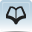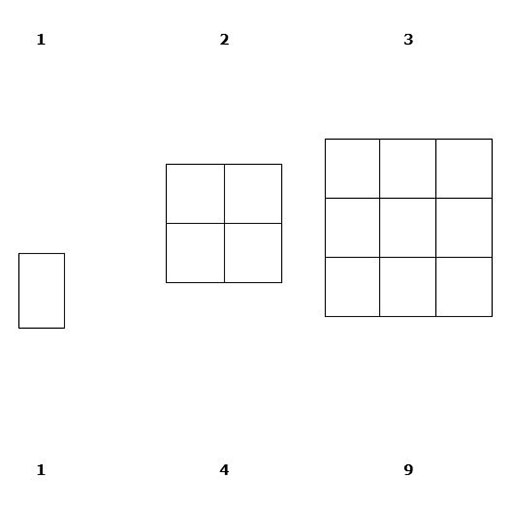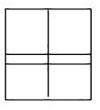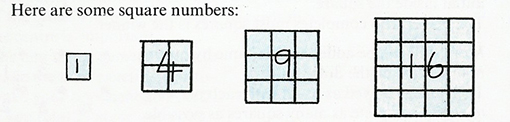# Resource 1: Square numbersTeacher resource for planning or adapting to use with pupils• First draw a square on the chalkboard labelling the sides 2 cm and divide it as shown into 1 cm squares.• The first recorder has to count the 1 cm squares and write down the number.
• The first scribe has to draw a 3 cm square and divide it similarly into 1 cm squares and the recorder will count and record these small squares.
• This activity continues with a 4 cm square, a 5 cm square, a 6 cm square.

## ExamplesSequence counting
• Ask the pairs to draw other square numbers to a hundred. The pairs take turns to draw and count/record the numbers.

3. Looking for patterns

Resource 2: Table of multiplications